# Chapter 14 Quadrilaterals RD Sharma Solutions Exercise 14.2 Class 9 Maths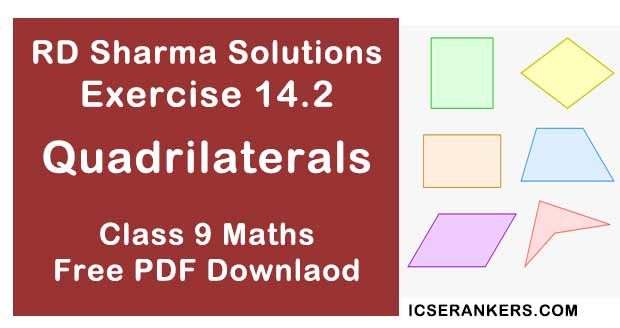Chapter Name RD Sharma Chapter 14 Quadrilaterals Exercise 14.2 Book Name RD Sharma Mathematics for Class 10 Other Exercises Exercise 14.1Exercise 14.3Exercise 14.4 Related Study NCERT Solutions for Class 10 Maths

### Exercise 14.2 Solutions

1. Two opposite angles of a parallelogram are (3x - 2)° and (50 - x)° . Find the measure of each angle of the parallelogram.

Solution

We know that
Opposite sides of a parallelogram are equal
∴ 3x  2 = 50 - x
⇒ 3x + x = 50 + 2
⇒ 4x = 52
⇒ x = 13°
∴ (3x - 2)° = (3 × 13 - 2) = 37°
(50 - x)° = (50 - 13°) = 37°
Adjacent angles of a parallelogram are supplementary
∴ x + 37 = 180°
∴ x = 180° - 37° = 143°
Hence, four angles are : 37°, 143°, 37° , 143°

2. If an angle of a parallelogram is two - third of its adjacent angle, find the angles of the parallelogram.

Solution

Let the measure of the angle be x
∴ The measure of the angle adjacent is 2x/3
We know that the adjacent angle of a parallelogram is supplementary
Hence x + 2x/3 = 180°
2x + 3x = 540°
⇒ 5x = 540°
⇒ x = 108°
⇒ x + 108° = 180°
⇒ x = 180° - 108° = 72°
⇒ x = 72°
Hence, four angles are :  180° , 72°, 108°, 72°

3. Find the measure of all the angles of a parallelogram, if one angle is 24° less than twice the smallest angle.

Solution

Let the smallest angle be x
Then, the other angle is (3x - 24)
Now, x + 2x - 24 = 180°
3x - 24 = 180°
⇒ 3x = 180° + 24
⇒ 3x = 204°
⇒ x = 204/3 = 68°
⇒ x = 68°
⇒ 2x -24° = 2×68° - 24° = 136° - 24° = 112°
Hence four angles are 68°, 112°, 68°, 112°

4. The perimeter of a parallelogram is 22cm. If the longer side measures 6.5 cm what is the measure of the shorter side ?

Solution

Let the shorter side be x
∴Perimeter = x + 6.5 + 6.5 + x
22 = 2(x + 6.5)
11 = x + 6.5
⇒ x = 11 - 6.5 = 4.5 cm
∴ Shorter side  = 4.5 cm

5. In a parallelogram ABCD, ∠D = 135°, determine the measures of ∠A and ∠B.

Solution

In a parallelogram ABCD
So, ∠D + ∠C = 180°
135 + ∠C = 180°
⇒ ∠C = 180° - 135°
⇒ ∠C = 45°
In a parallelogram opposite sides are equal
∠A = ∠C = 45°
∠B = ∠D = 135°

6. ABCD is a parallelogram in which  ∠A = 70°. Compute ∠B, ∠C and ∠D.

Solution

In a parallelogram ABCD
∠A = 70°  [∵ Adjacent angles supplementary]
∠A = ∠B = 180°
⇒ 70° + ∠B = 180°  [∵ ∠A = 70°]
⇒ ∠B = 180° - 70° = 110°
In a parallelogram opposite sides are equal
∠A = ∠C = 70°
∠B = ∠D = 110°

7. In fig., below, ABCD is a parallelogram in which  ∠A = 60 . If the bisectors of ∠A and ∠B
meet at P, prove that AD = DP, PC = BC and DC = 2AD.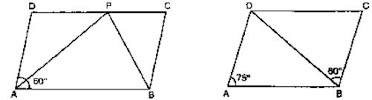Solution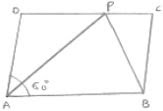AP bisects ∠A
Then, ∠AP  = ∠PAB = 30°
Then, ∠A + ∠B = 180°
∠B + 60° = 180°  [∠A = 60°]
⇒ ∠B = 180° - 60°
⇒ ∠B = 120°
BP bisects ∠B
Then, ∠PBA + ∠PBC = 30°
∠PAB = ∠APD = 30°  [Alternative interior angles]
∴ AD = DP  [∵ Sides opposite to equal  angles are in equal length]
Similarly
∠PBA = ∠BPC = 60°  [Alternative interior angle]
∴ PC = BC
DC = DP + PC
⇒ DC = AD + BC  [∵ DP = AD, PC = BC]
[∵ AD = BC Opposite sides of a parallelogram are equal].

8. In fig. below, ABCD is a parallelogram in which ∠DAB  = 75 and ∠DBC = 60° . Compute ∠CDB and ∠ADB.
Solution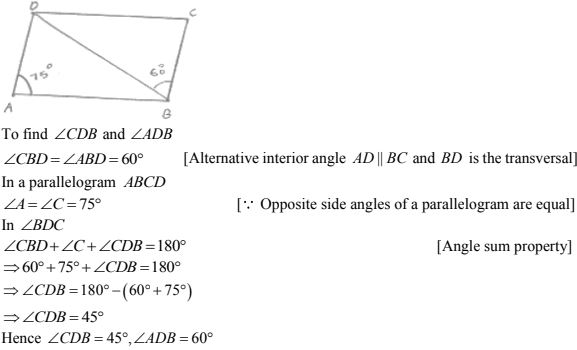9. In below fig. ABCD is a parallelogram and E is the mid - point of side BC. If DE and AB when produced meet at F, prove that AF = 2AB.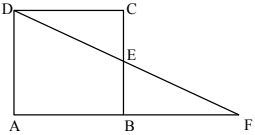Solution
In ΔBEF and ΔCED
∠BEF = ∠CED  [Verified opposite angle]
BE = CE  [∵ E is the mid - point of BC]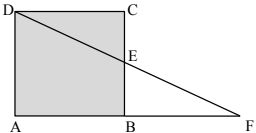∠EBF = ∠ECD   [∴ Alternate interior angles are equal]
∵ ΔBEF ≅ ΔCED   [Angle side angle congruence]
∵ BF = CD  [Corresponding Parts of Congruent Triangles]
AF = AB + AF
AF = AB + AB
AF = 2AB

10. Which of the following statements are true (T) and which are false (F)?
(i) In a parallelogram, the diagonals are equal.
(ii) In a parallelogram, the diagonals bisect each other.
(iii) In a parallelogram, the diagonals intersect each other at right angles.
(iv) In any quadrilateral, if a pair of opposite sides is equal, it is a parallelogram.
(v) If all the angles of a quadrilateral are equal, it is a parallelogram.
(vi) If three sides of a quadrilateral are equal, it is a parallelogram.
(vii) If three angles of a quadrilateral are equal, it is a parallelogram.
(viii) If all the sides of a quadrilateral are equal it is a parallelogram.
Solution
(i) False
(ii) True
(iii) False
(iv) False
(v) True
(vi) False
(vii) False
(viii) True